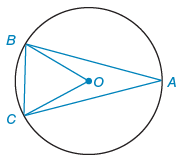Alyssa Z.

# Given:  ⊙O with AB ≅ AC m∠BOC = 84°.Find the following in degrees.

Consider the following figure.A circle has center point O. There are 3 points on the circle and 5 interior line segments.

1. Point A is on the right.
2. Point B is on the upper left.
3. Point C is on the lower left.
4. Two segments extend from A: segment A B lies above O, and segment A C lies below O.
5. Two segments extend from O: segment O B and segment O C.
6. Segment B C goes from B down to C.
 Given: Given:    ⊙O with AB ≅ AC m∠BOC = 84°

Find the following in degrees.

(a) mBC°

(b) mAB°

(c) m∠A

°

(d) m∠ABC

°

(e)m∠ABO

By:

Alyssa Z.

Would it ABO be 84?
Report

24dJon S.

tutor
ABC is 69 so ABO wouldn't be 84. I was thinking that maybe OB bisects ABC so that ABO would be 34.5, but I am not sure so I did not put that down as an answer.
Report

24d

## Still looking for help? Get the right answer, fast.

Get a free answer to a quick problem.
Most questions answered within 4 hours.

#### OR

Choose an expert and meet online. No packages or subscriptions, pay only for the time you need.